# Triangulation

Why quality?

Quotation from Deming

Knowledge of variation: the range and causes of variation in quality, and use of statistical sampling in measurements; Improve constantly and forever the system of production and service, to improve quality and productivity, and thus constantly decrease costs. Break down barriers between departments. People in research, design, sales, and production must work as a team, to foresee problems of production and usage that may be encountered with the product or service. In god we trust, everybody else bring data

## Cell coverage

We take one locationexample of a location

We download the local street network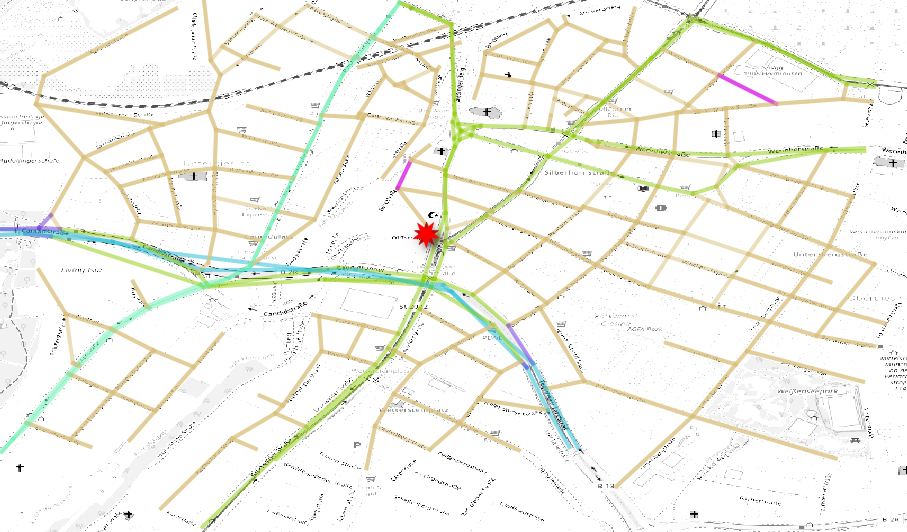local network

We download the cell coverage in the network area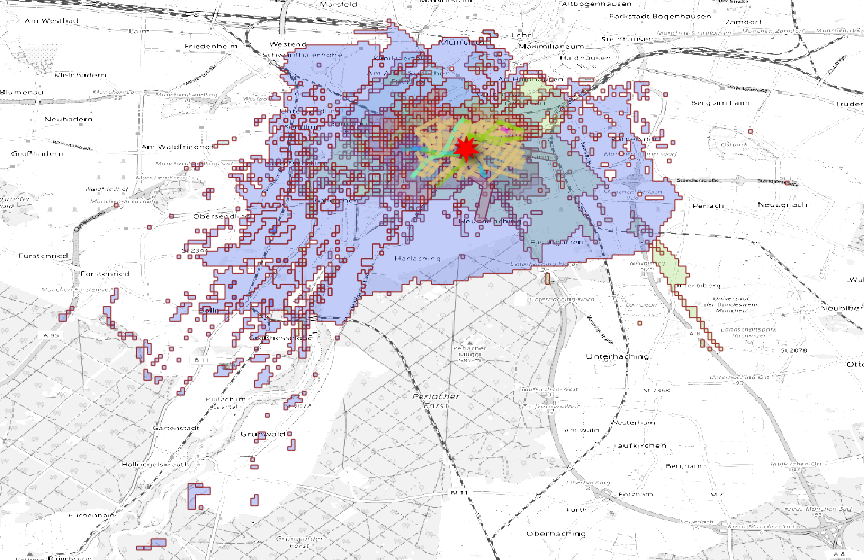cell coverage around the location

We classify the cells by radius and we plot the coverage centroid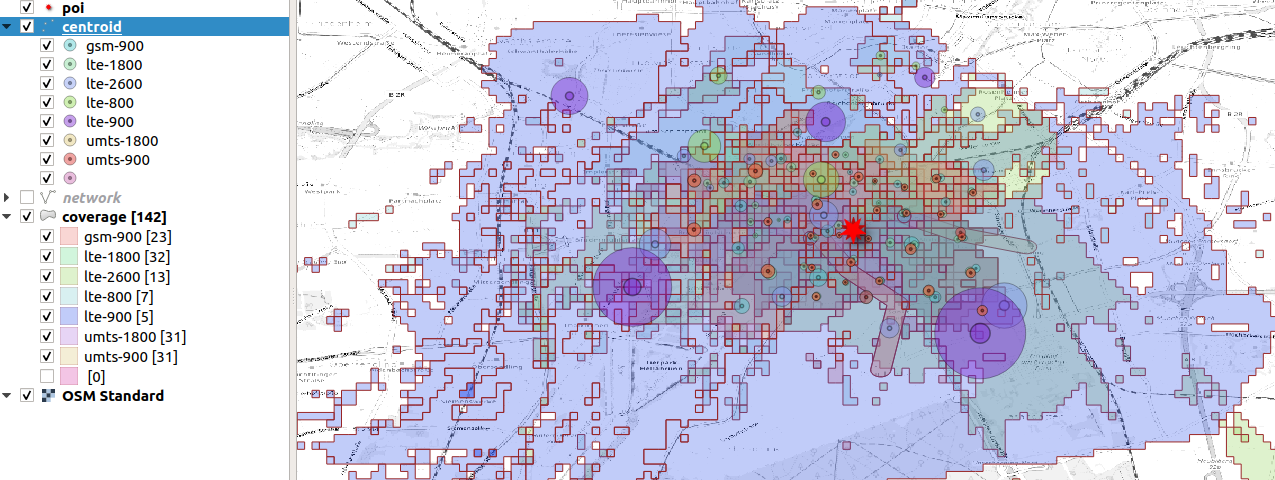display cells by technology wich influences the area

We select the most precise cells, some of them happen to be subway cells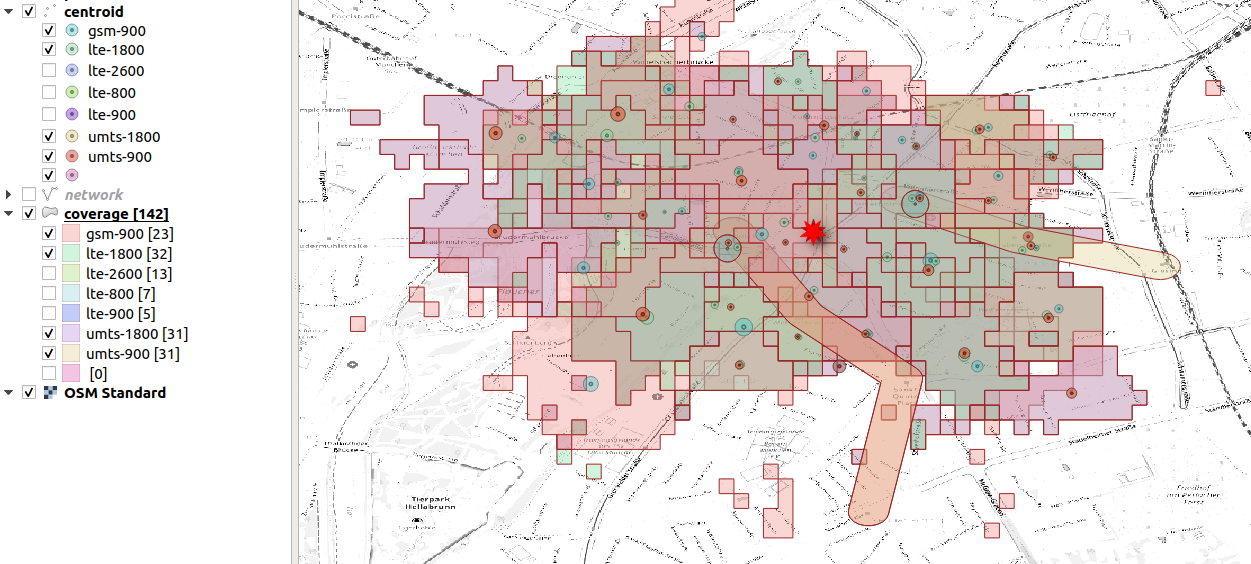selection of the techologies with good spatial resolution

We can simplify the geometries taking only the closest and most precise cells centroidsneighboring cells represented by centroid and radius

and than we try to sum up a portion of activities into some geometrieswe map the location into a geometry (mtc, zip5)

But for some technology we have centroids outside the coverage area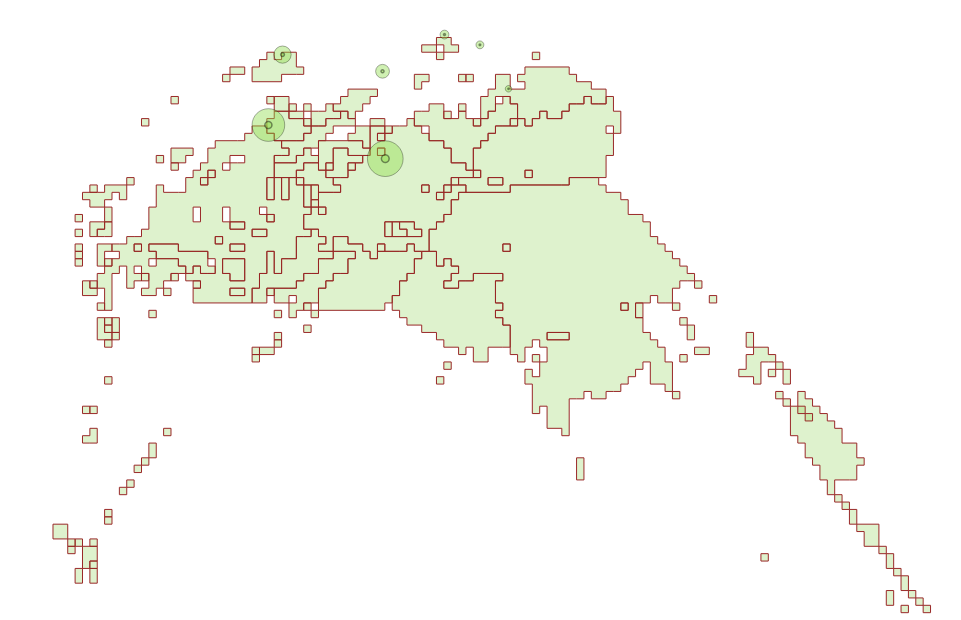some centroids are not contained in any area

## Stability comparison

We want to evaluate count stability using the dominant cell method.

The first test is done moving the center of the overlapping mapping by a displacement (in meters)stability of counts after displacement

We than check the stability of counts by area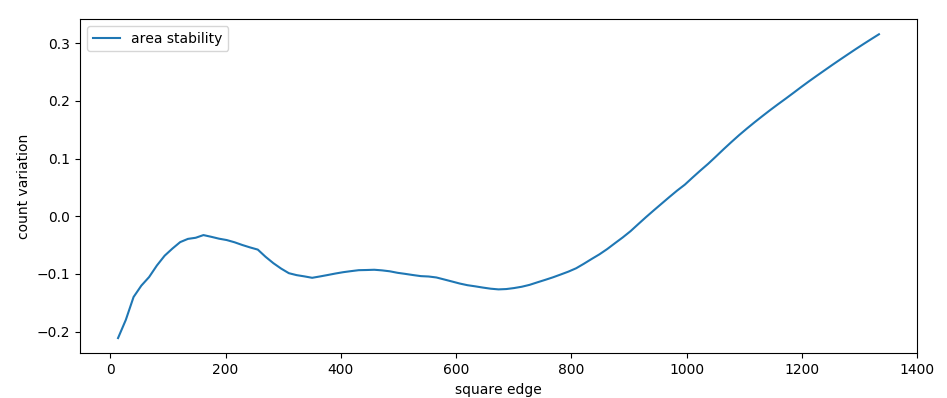stability of counts after area increase (edge in meters)

Number of counts strongly increase with mapping area (until 50%).

## definition

An activity is made of many events. Currently we define the dominant cell as the cell where most of the events took place. For every cell we assign a position which is the centroid of the BSE (Best Server Estimation), an estimation of the area where the cell has the best ground coverage without intersection of other cells with the same technology. Hence, each activity position is located at the centroid of each dominant cell.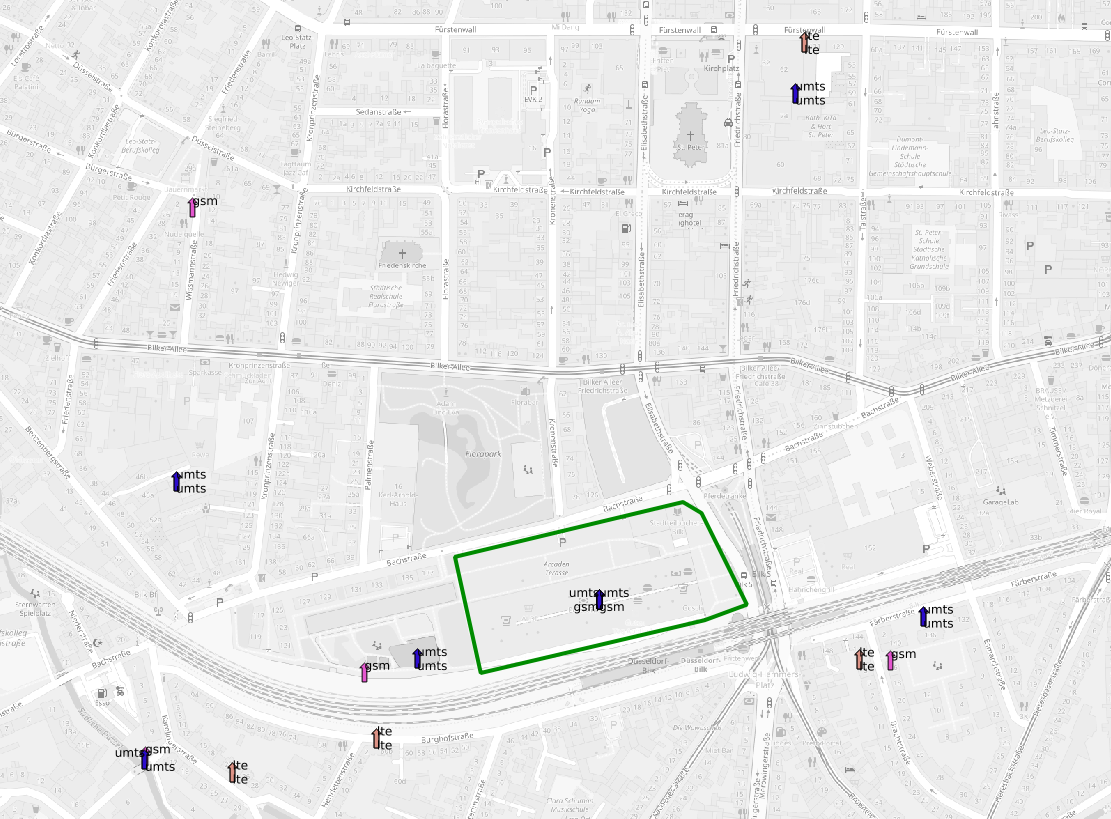The polygon shows the location we want to isolate, the arrows the neighbouring cell centroids

The density profile of the activities is then centered around the centroid position.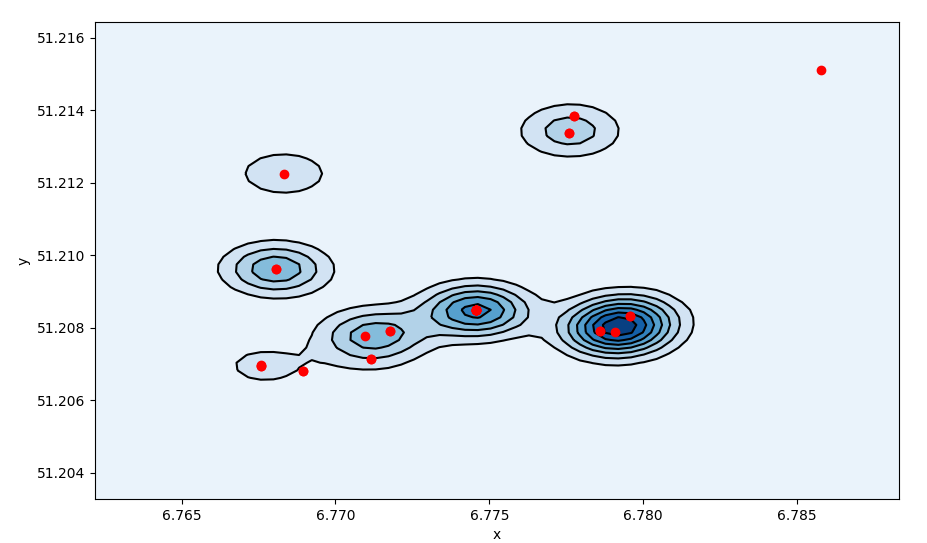We can divide the space where each cell centroid sits at the vertex of a Delaunay triangle.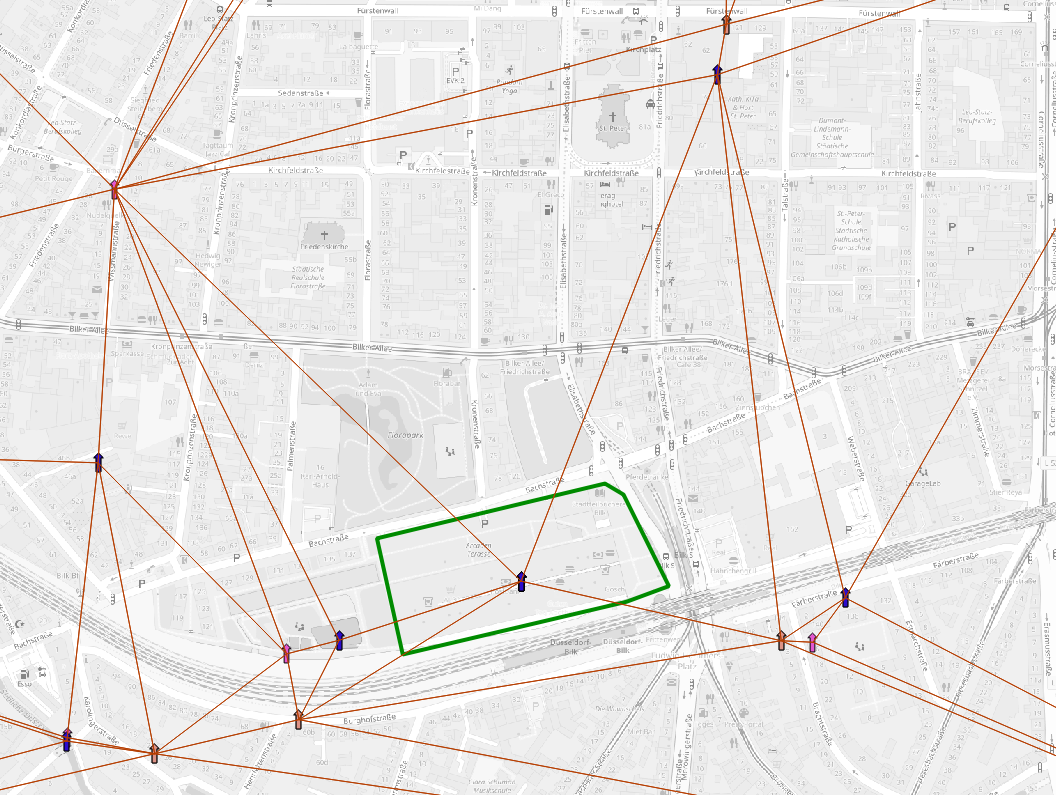Delaunay triangulation on cell centroids

### Triangulation

If we average over all vertex positions we have a more sparse activity distribution. We can even define a standard error for each activity filter_activities.py.
$$s_x = \sqrt{\frac{ \sum_i (\hat x - x_i)^2 }{N-2}} \qquad s_r = \sqrt{s_x^2 + s_y^2}$$
The standard error define the uncertanty on the activity position.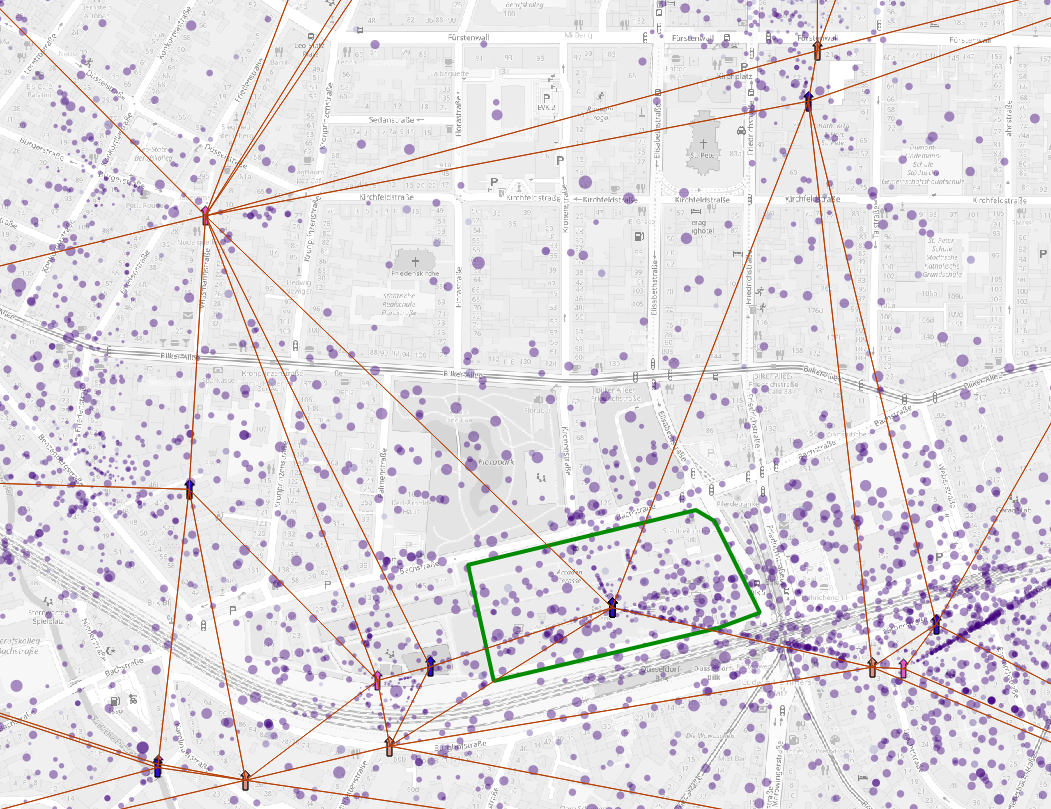The dots represent the average activity position, the dot size represent the standard error of the position

Which translate in a broader density profile.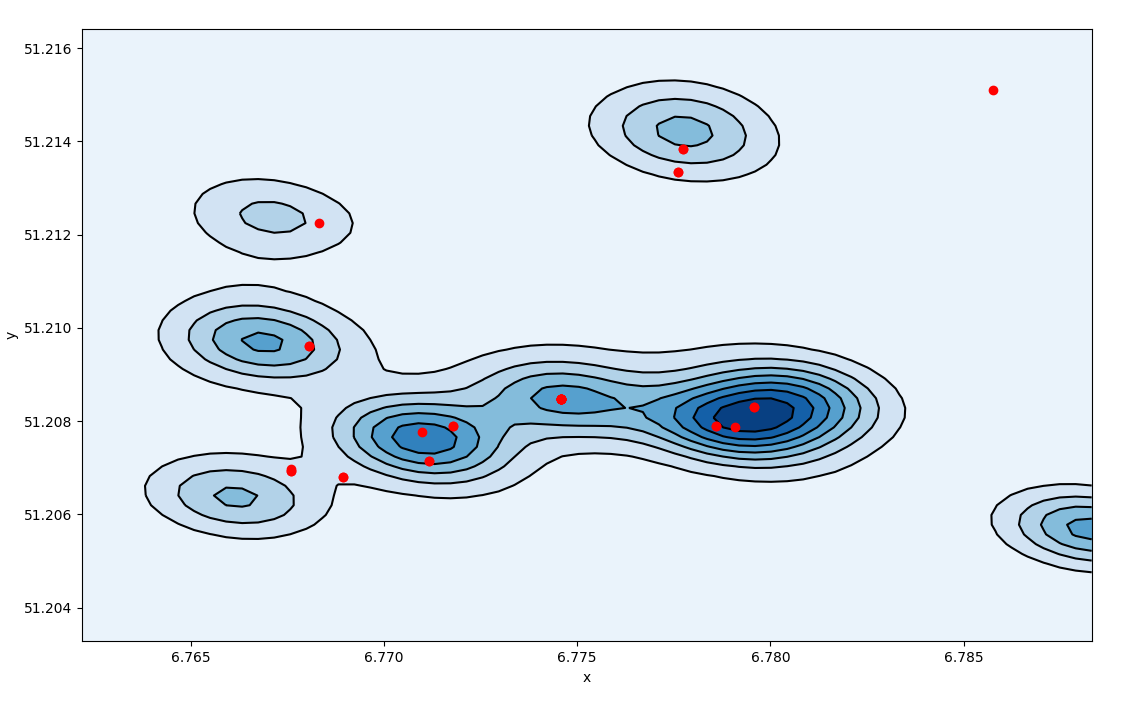Density profile of triangulated activities, density spreads over the space

For each polygon we take the distribution of standard error of the positions inside the polygon.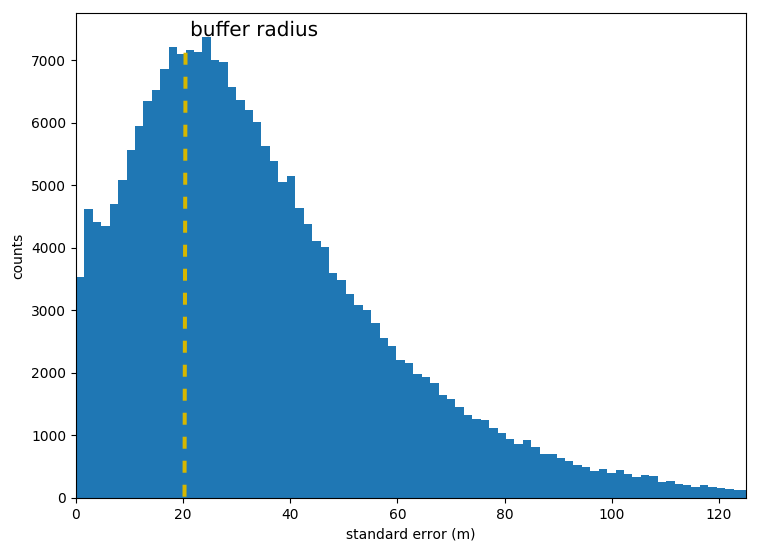distribution of the standard errors all over the activities

The median of the standard error distribution defines a buffer radius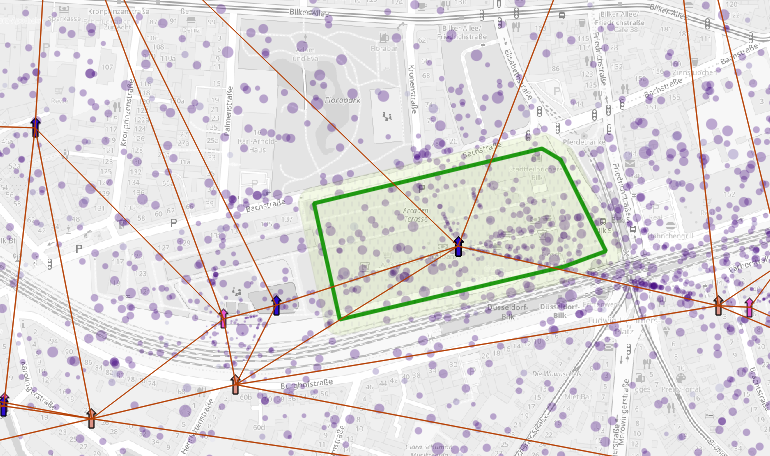The buffer radius (in green) circulates around the polygon defining the precision of activity positions per location

### Octree binning

To overcome anonymization problems we transform coordinates in geohash.

Geohash is a indexed short string which contains information about the position and the bounding box of a square. The number of digits sets the precision (i.e. the size of the bounding box) using a octree. Neighbouring boxes have similar indices.2d representation of an octree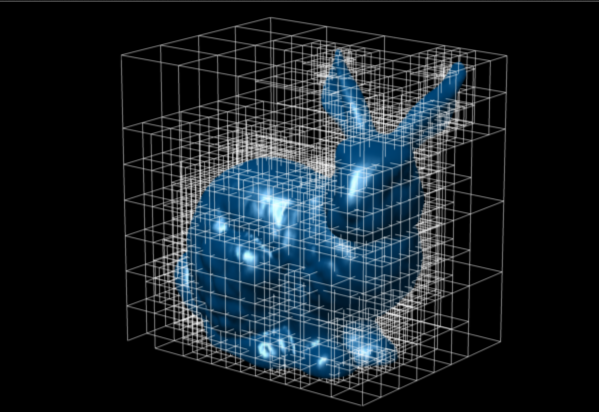3d usage of an octree

To anonymize the data we loop until we find the minimum number of digits for each geohash string: fenics_finite.py.

vact.loc[:,"geohash"] = vact[['x_n','y_n']].apply(lambda x: geohash.encode(x,x,precision=8),axis=1)
def clampF(x):
return pd.Series({"n":sum(x['n']),"sr":np.mean(x['sr'])})
lact = vact.groupby('geohash').apply(clampF).reset_index()
for i in range(3):
setL = lact['n'] < 30.
lact.loc[:,"geohash2"] = lact['geohash']
lact.loc[setL,"geohash"] = lact.loc[setL,'geohash2'].apply(lambda x: x[:(8-i-1)])
lact = lact.groupby('geohash').apply(clampF).reset_index()

At the end we produce a table similar to this one:

geohash count deviation
t1hqt 34 0.1
t1hqt3 42 0.4
t1hqt3zm 84 0.8
t1hqt6 44 0.6
t1hqt8 78 0.8
t1hqt9 125 0.6
t1hqt96 73 0.3
t1hqt97 38 0.5
t1hqt99 46 0.4

In post processing we can then reconstruct the geometry: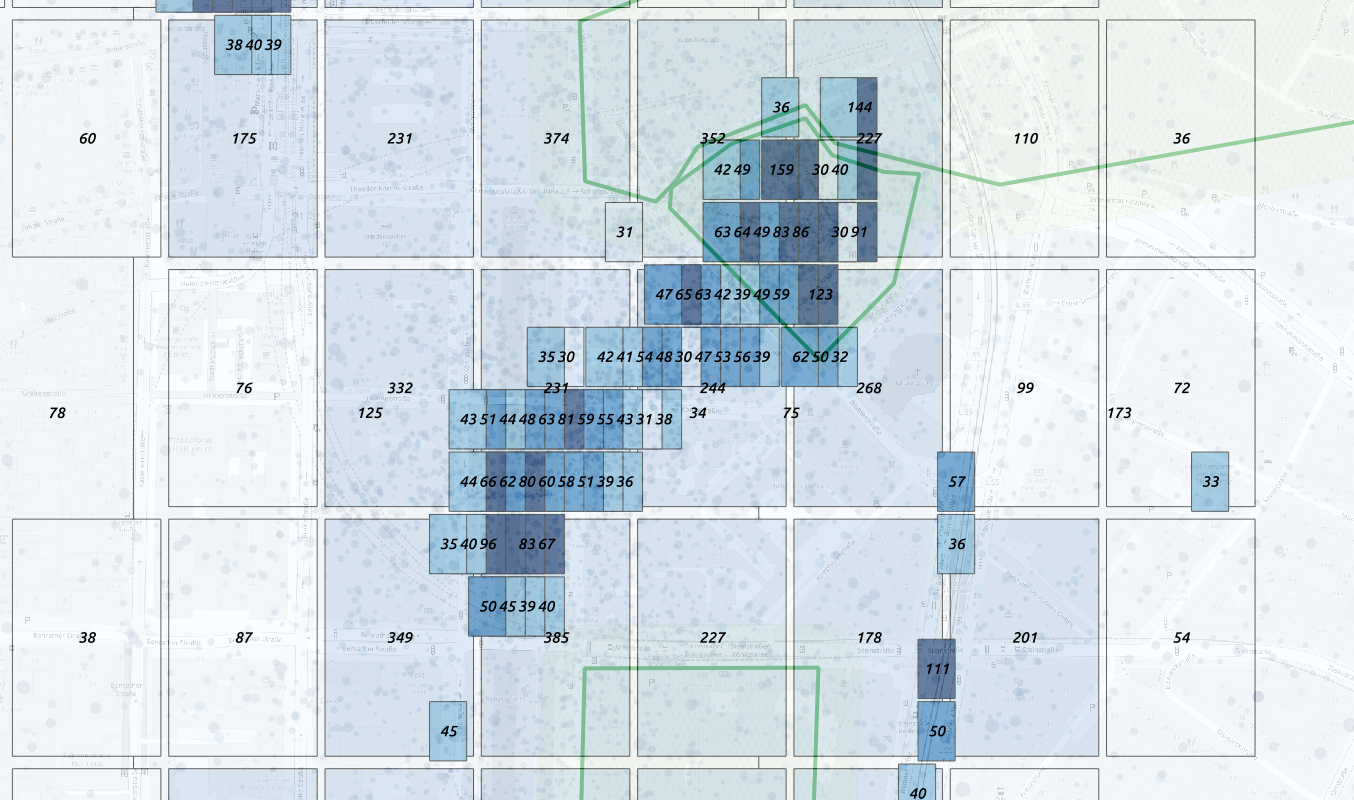Overcome the anonimization problem using geohash binning

This allows us to have the larger precision allowded without count loss.

For each box we can estimate the precisionOvercome the anonimization problem using geohash binning

Alternatively we can apply a double binary octree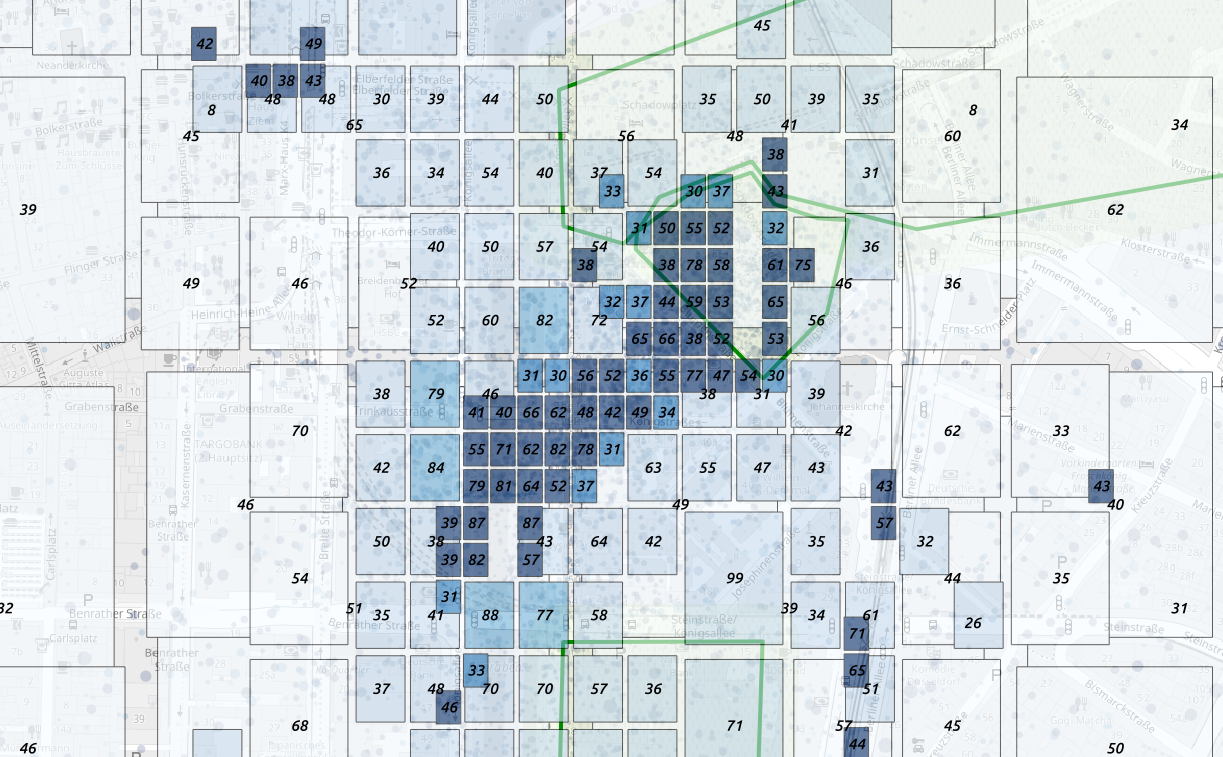counts on a octree binning

Advantages * no mapping * no BSE * no data loss * custom geometries (postprocessing - no deploy) * no double counting * highest allowed resolution

Cons * no unique counts per polygon * postprocessing always required * land use mapping might have more accuracyevolution of mappings

Which was calculated by a simple halfing of each edge:

   BBox = [5.866,47.2704,15.0377,55.0574]
spaceId = ''
for i in range(precision):
marginW = marginH = 0b00
dH = (BBox - BBox)*.5
dW = (BBox - BBox)*.5
if x < (BBox + dW):
BBox = BBox - dW
else:
marginW = 0b01
BBox = BBox + dW
if y < (BBox + dH):
BBox = BBox - dH
else:
marginH = 0b10
BBox = BBox + dH
spaceId = spaceId + str(marginW + marginH)

In post processing we can reconstruct the geometry and interpolate the missing boxes.

### Space deformation

The spatial resolution is given by the distribution of cells which modifies the definition of space around. We represent each cell centroid as a trapezoid whose center corresponds to the cell centroid and the area to a portion of the BSE area fenics_finite.py. We define an Helfrich Hamiltonian on a manifold: z = z(x, y) to define the curvature of space caused by the cell position:
$$F(z) = \frac{1}{2} \int d x d y (k_{ben}(\nabla^2 z)^2 + k_{stif} (\nabla z)^2 )$$
Where the bending and stiffness parameters are controlled by the parameters kben and kstif.

To solve the equation we chose a square mesh and we apply finite differences method on the lattice representing the space. The solution of the equation is done numerically applying a Jacobi iteration on a 5x5 square matrix to convolve with the square lattice representing the space. kben and kstif represent the weights for the Laplacian Δ and the square Laplacian term Δ2.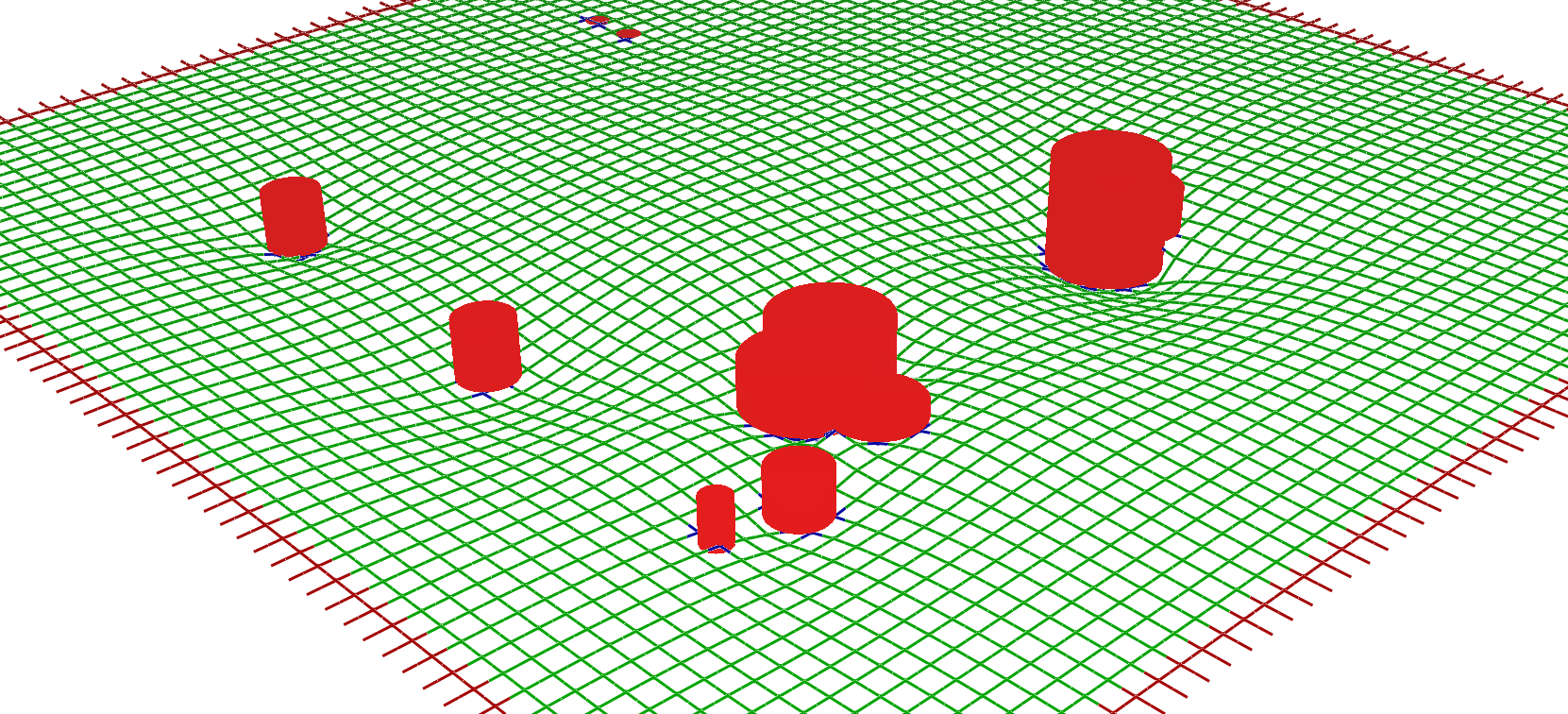Solution of the Helfrich Hamiltonian on a square lattice, the cylinders represent the cell centroids The boundary conditions and the constraints are given by the red and blue beads which are not moved during the iterations. We can increase the resolution interpolating the lattice points.discretization of the lattice solution

To normalize the effect of the space curvature on the activity positions we move each activity by the gradient of the space deformation
$$\nabla z = \frac{\partial z}{\partial x} \hat x+ \frac{\partial z}{\partial y} \hat y$$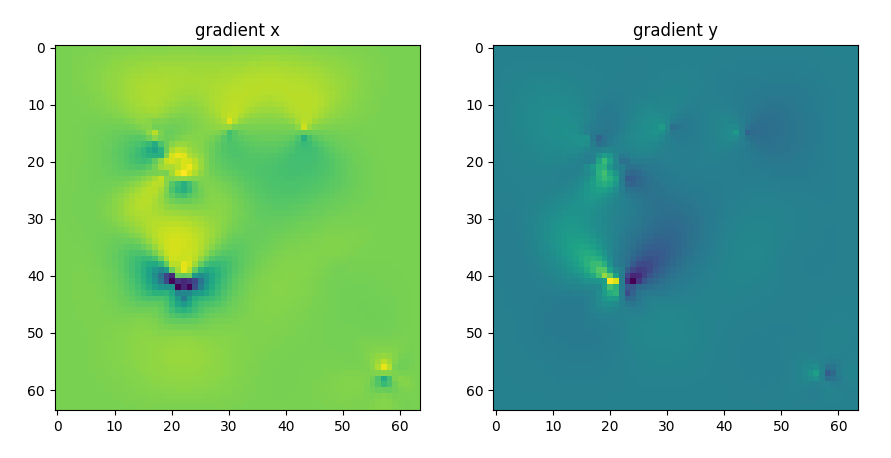gradient of the space deformation on the x and y directions

If we invert the gradiant we obtain the displacement induced by the cell geography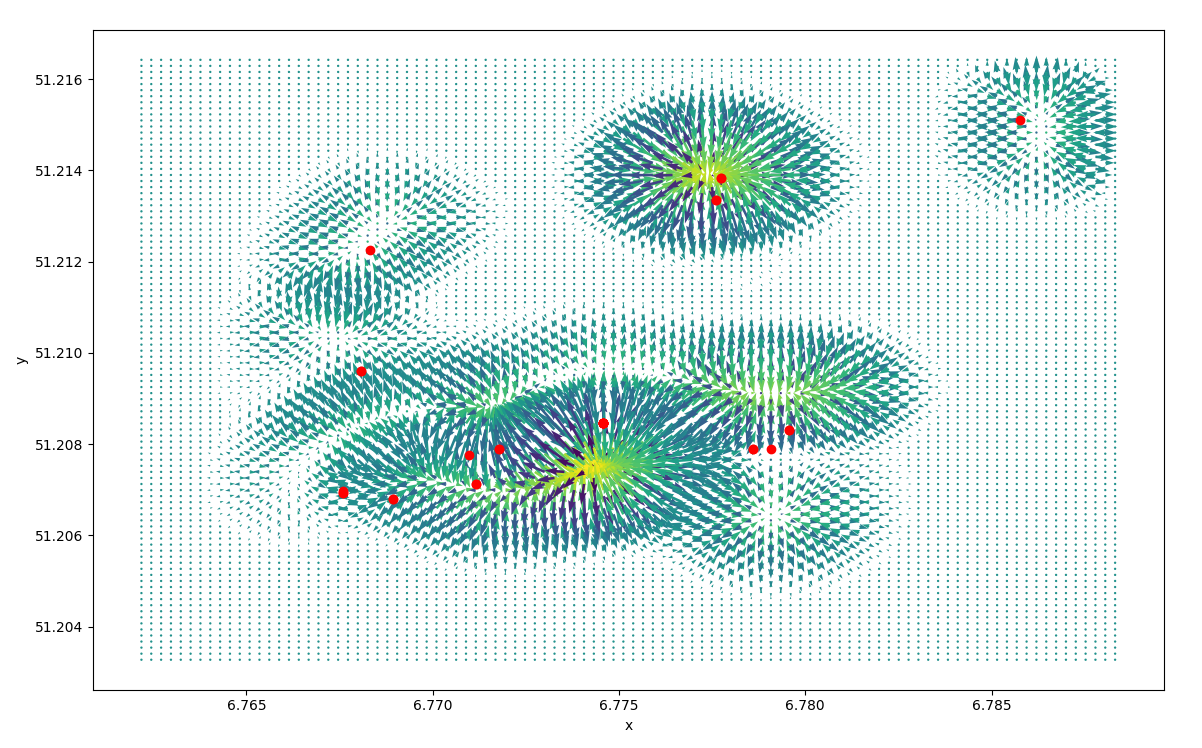vector field of the displacement and centroids

We can see how the single activities have moved.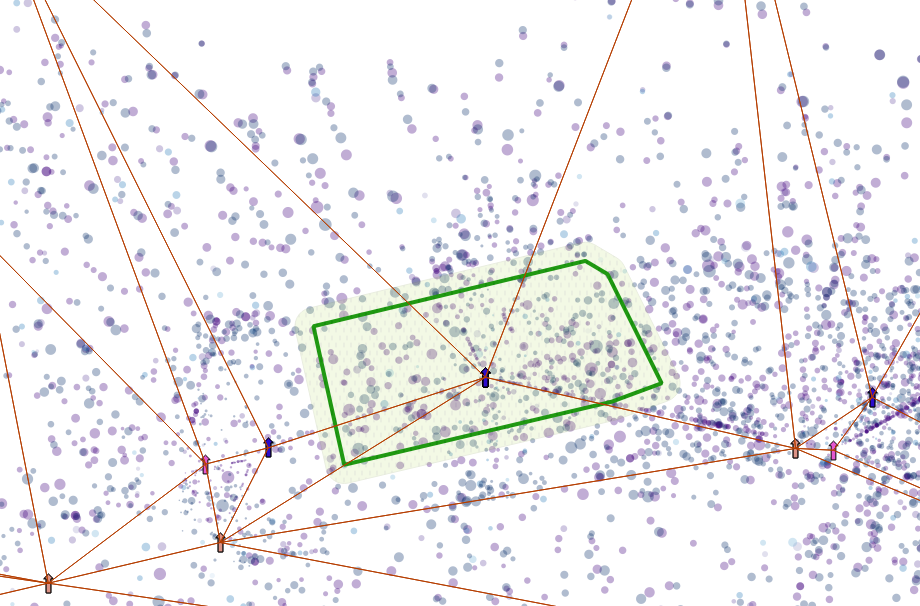shift of activities, purple to blue

We then recalculate the density plot based on the new positions.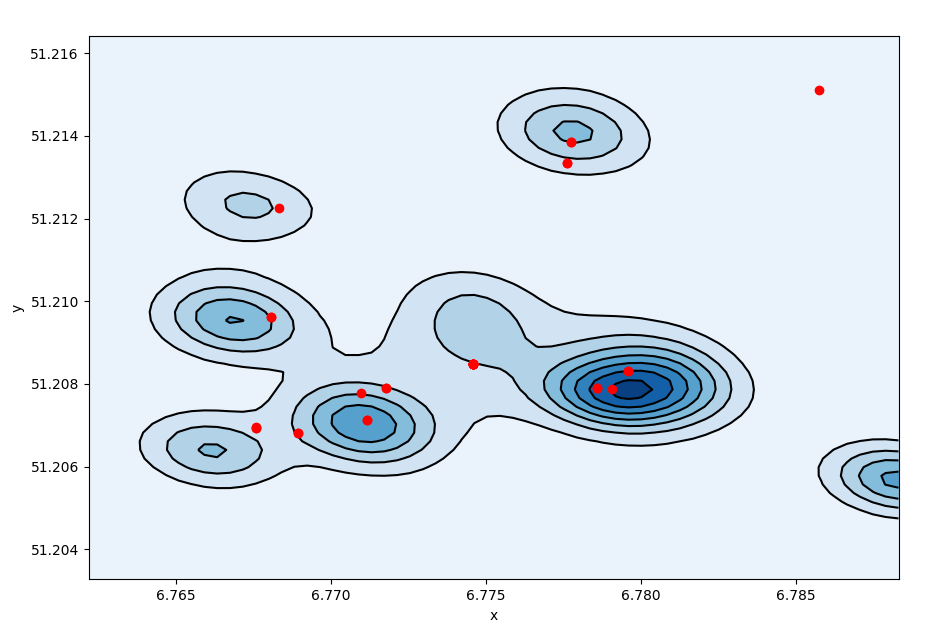density plot after displacement

We move the single activities according to the gradient.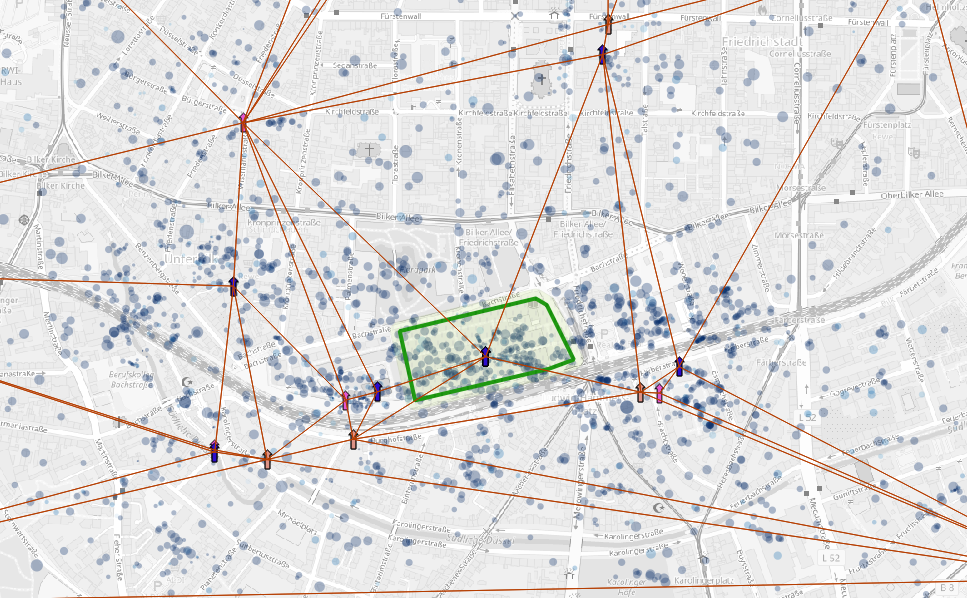new activity position after displacement

On top of the cell space distortion we apply the underlying network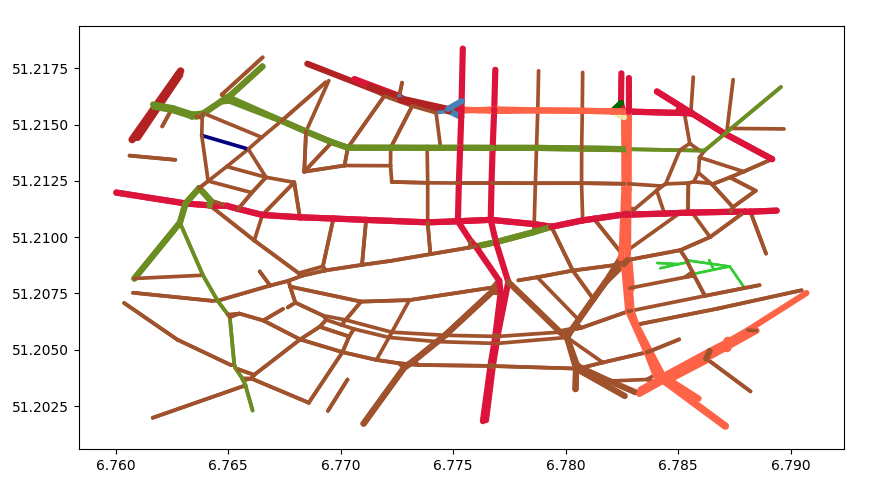underlying netwok, each street class has a different color

We discretize the network, apply a width depending on the street speed and apply a smooting filter.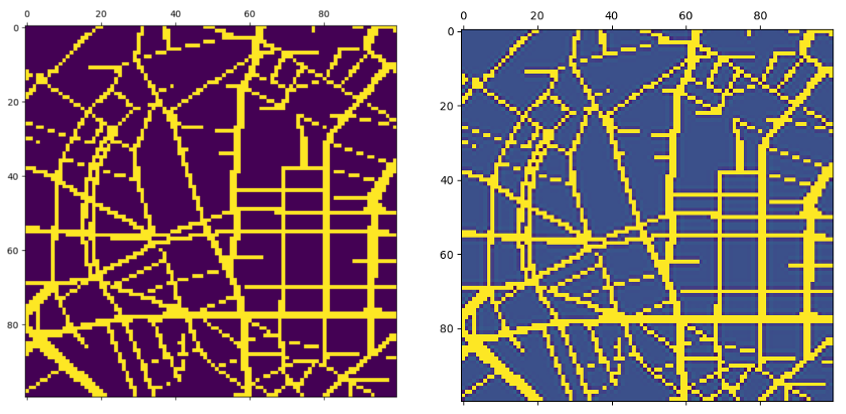smoothed filter on network

Similarly we can create a displacement map based on the street network.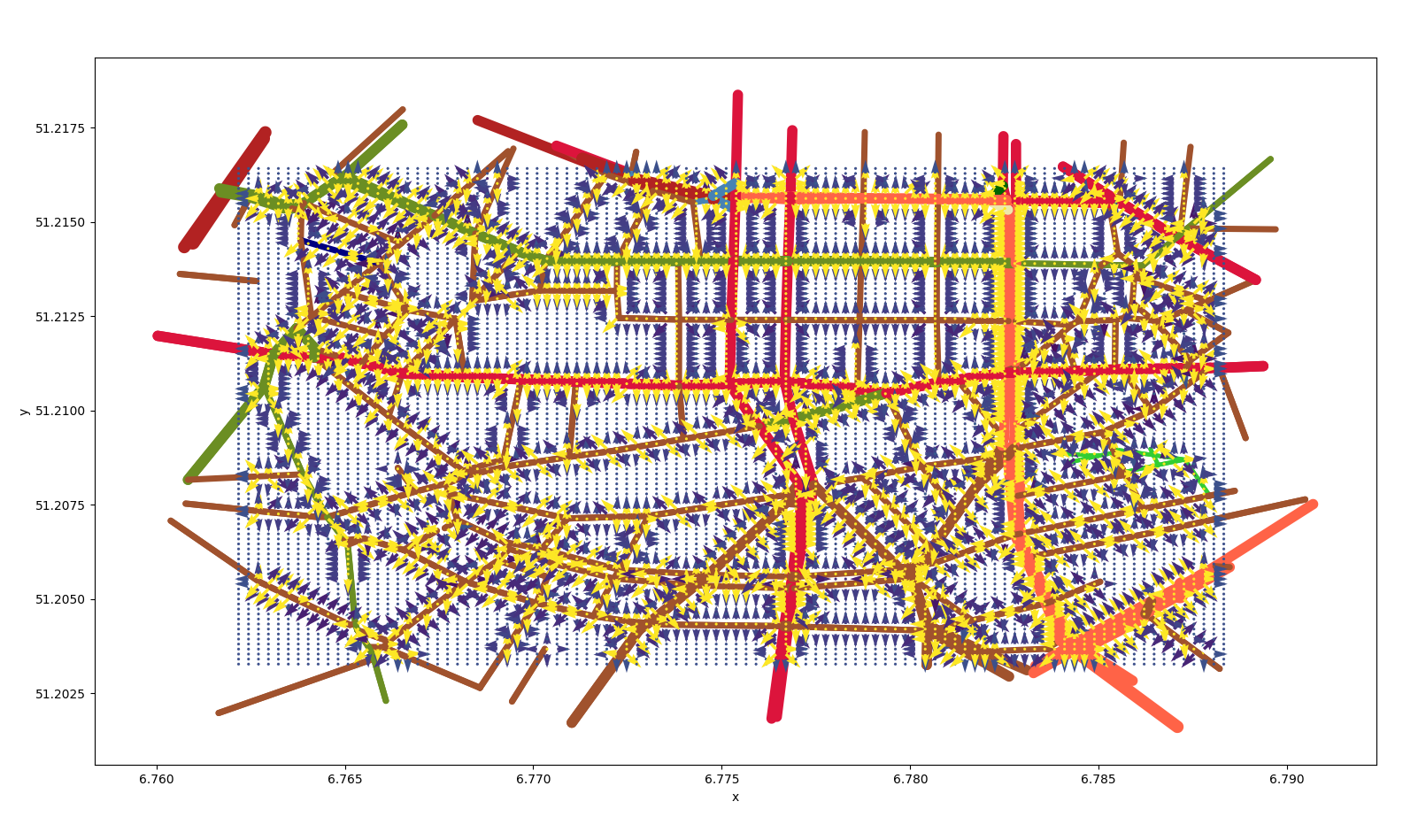displacement due to the street network

To summarise the whole scheme of the procedure is described in this scheme: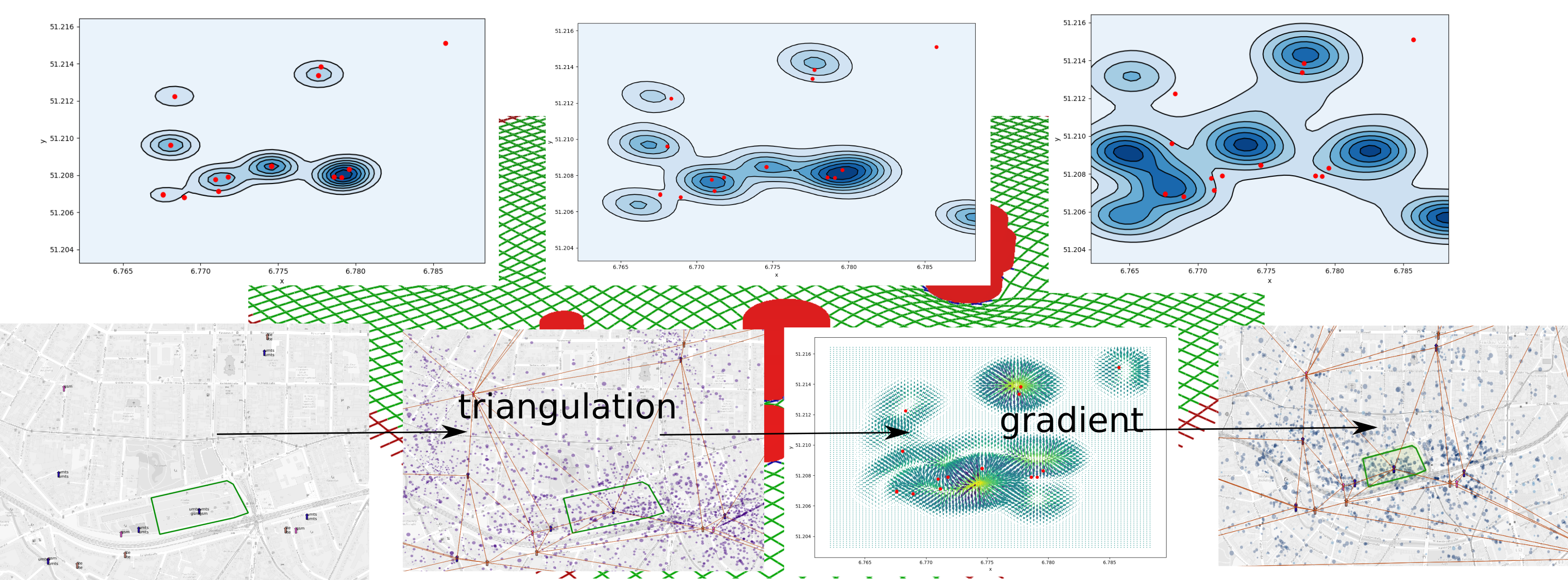density plot after each operation and overview on the procedure

The procedure show how to create a more realistic density plots. Further tuning is necessary.

Finally we update the mapping etl_roda.py.